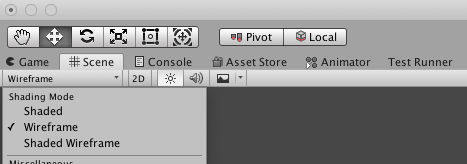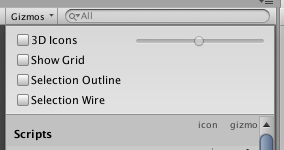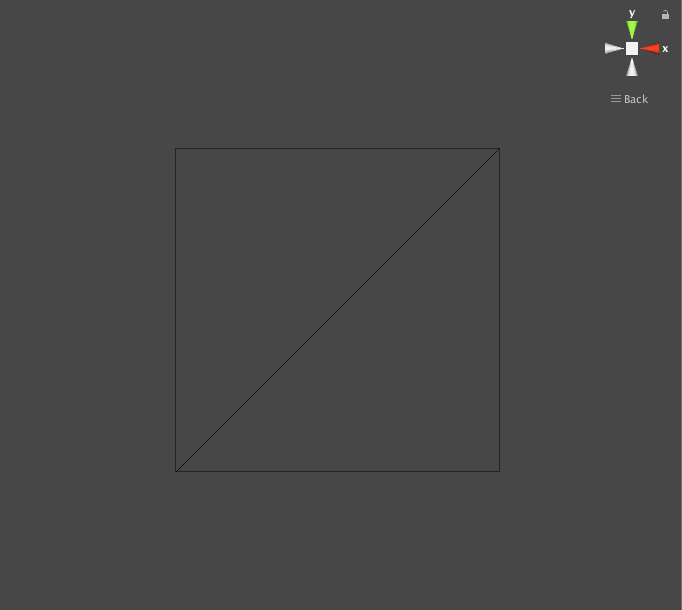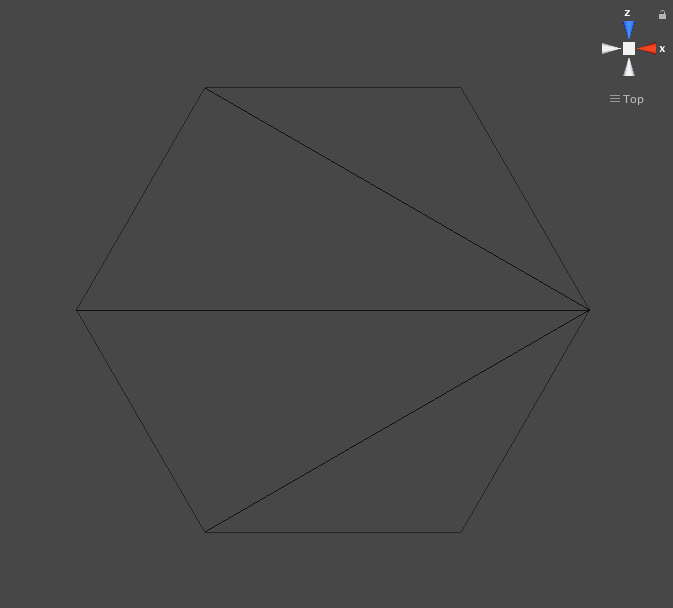# Meshes

I often resort to placeholder assets when putting together prototype projects. Unity’s built-in selection of primitive shapes like the Cube, Quad, Sphere and Capsule are very useful toward that purpose. More often that not, any other assets you work with will be created externally and then imported into Unity. This is especially true with something as complex as a 3D mesh. However, there are reasons to create and/or edit meshes programmatically, and this lesson will serve as a helpful introduction.

## Areas Of Use

In the past I have used procedurally generated meshes for a variety of purposes. Procedural level generation (like mazes) and UI elements (with fancy stuff like shearing) are some of the more notable jobs. I also use it to export mocked levels from my prototypes to actual 3D Modeling apps to use for reference or to build on top of.

Most recently, I was mocking up a board game for myself. The game was designed around a hex board. Unity offers a nice quad object, but no hexagon. Unity also doesn’t allow control over the number of segments in its cylinder, so I couldn’t cause it to appear as a hex shape. I needed another solution. I could have created a simple hex in a 3D engine and imported it into Unity, but this is actually not the first time I have wanted a hex, so I thought it might be nice to have as a Unity script instead. In fact, this lesson will show how I built that script so you can use it too.

## Getting Started

Open Unity and create a new project. I named mine “BlogMesh”, not that the project name matters. Next I created a new “Scripts” folder, as well as our first and only script for this tutorial, “HexMeshWindow”, which was placed inside.

We will be creating an “EditorWindow” which is like our own tool to use inside the Unity editor. This means we will need to add another “using” statement:

```using UnityEditor;
```

And then we will modify the class definition so that it inherits from “EditorWindow”:

```public class HexMeshWindow : EditorWindow
```

Unity has a special tag called “MenuItem” with which you can mark your code. This lets me add items to Unity’s menu bar. The action of selecting the menu item will be to invoke the method that was marked by the tag. We will use this opportunity to create a new floating window which will have the interface options we want in order to create our mesh. Add the following code inside the body of our new class:

```[MenuItem("Window/Hex Mesh creator")]
static void Init()
{
var window = (HexMeshWindow)EditorWindow.GetWindow(typeof(HexMeshWindow));
window.Show();
}
```

If you save and build your code, you can actually start trying it out. Head back to Unity, then look in the menu bar under: “Window -> Hex Mesh Creator”:After selecting the menu item, a new window labeled “HexMeshWindow” should open. It will be empty, but we will add content soon. You can close it for now.

This is going to be a pretty simple project. All I want to add is an input field to allow me to control the radius of the hex shape, and a button to actually create the mesh. In order for the window to store and work with the input radius, I’ll add a float variable. The UI elements will be created by Unity via the “EditorGUILayout” and “GUILayout” elements they provide. This will be put together inside the “OnGUI” method.

```float radius = 0.5f;

private void OnGUI()
{
if (GUILayout.Button("Create"))
{
CreateMesh();
}
}

private void CreateMesh()
{

}
```

For now, I have merely added a stub for “CreateMesh” which will be invoked whenever a user clicks the “Create” button in the interface. We will fill it out later, after explaining how meshes are put together. Until then, feel free to open your window again, and you should see something like the following:## Meshes

Now I’d like to take a quick detour to explain how meshes are put together. There are four important elements to cover: vertices, triangles, uvs and normals. For a visual, try creating a Quad in Unity via the menu bar: “GameObject -> 3D Object -> Quad”. Look in the “Scene” tab, set the shading mode to Wireframe:If you like, you can also uncheck “Show Grid” from the Gizmos pull down (also on the Scene window) which may make it a little easier to see the geometry of the mesh.After focusing on the quad and rotating the view to look straight at it, you should see something like this:Now we can see two of the elements of our mesh: vertices and triangles. There are four vertices – one at each corner of the quad. You can also see that there are two visible triangles which create the surface of the shape.

A vertex is just a point in 3D space and it can be represented by a “Vector3” struct. A triangle is a group of three vertices that define a plane in 3D space. They are defined by an array of integers, where each integer is the index of one of our vertices.

For ease of explanation, imagine that the points are created clockwise, starting from the bottom left. The order of their creation or index in the array is irrelevant to the mesh itself though. When centered around the origin, the vertices of this mesh might be defined as:

```// Demo code only, don't add to HexMeshWindow script
mesh.vertices = new Vector3
{
new Vector3(-0.5f, -0.5f, 0),   // Bottom Left
new Vector3(-0.5f, 0.5f, 0),    // Top Left
new Vector3(0.5f, 0.5f, 0),     // Top Right
new Vector3(0.5f, -0.5f, 0)     // Bottom Right
};
```

Unlike the order of the vertices, the order of the indices for your triangles is actually important. Each set of three indices defines a triangle, and the order you list the vertices controls the winding-order of the polygon. The winding order is what controls which side of the polygon is visible. In Unity’s scene view you can see this effect by rotating the quad around. From behind, the quad is invisible (this is true in wireframe as well as shaded display modes). So, we will also select our vertices for each triangle in a clock-wise order so that they will face the camera. This could be defined as:

```// Demo code only, don't add to HexMeshWindow script
mesh.triangles = new int
{
0, 1, 2,    // Top triangle
0, 2, 3     // Bottom triangle
};
```

A mesh’s uv’s are somewhat similar to its vertices. There will be one uv point for each vertex. The vertices control the mesh’s position in 3D space, but the uv’s control the mesh’s postion in texture space. 3D space is basically unbounded, but texture space is usually normalized with value ranges from (0,0) for the lower left corner of the texture to (1,1) for the upper right corner of the texture. Our texture space is also defined in 2D, so we will use “Vector2D” for each uv. Assuming we would want a texture to map its full extents across the surfaces of our mesh, this could be defined as:

```// Demo code only, don't add to HexMeshWindow script
mesh.uv = new Vector2
{
new Vector2(0, 0),  // Bottom Left
new Vector2(0, 1),  // Top Left
new Vector2(1, 1),  // Top Right
new Vector2(1, 0)   // Bottom Right
};
```

The final element of our mesh is its normals. There will be one normal for each vertex. However, unlike vertices which are “points” that determine a location in space, our normals are “vectors” that determine a direction in space. They are used in controlling how light is reflected off the surface of the mesh. Generally speaking, each normal should represent a direction that is perpendicular to the plane of the triangle it is attached to. If the same vertex is used on multiple non-planar triangles, then the resulting normal could be a blend of the perpendicular angles. For our quad, both triangles occupy the same plane and so we can easily determine the direction they should face. They could be defined as:

```// Demo code only, don't add to HexMeshWindow script
mesh.normals = new Vector3
{
Vector3.back,   // Bottom Left
Vector3.back,   // Top Left
Vector3.back,   // Top Right
Vector3.back,   // Bottom Right
};
```

## Create the Hexagon

Now that we have our setup and a basic understanding of mesh components, let’s put it all together and finish our little project! But… how do we know the values for our points? It’s not as obvious as plotting points for a quad, especially if we want control over the radius.

Well, its actually not that hard if you have a little trigonometry under your belt. We are going to use the sine and cosine functions to help plot our circle. If you’ve never seen this before, here are a couple of quick resources to help you visualize what is happening:

Note – our quad mesh was created along an XY plane like Unity’s native quad. The hex mesh we will be creating will be plotted along the XZ plane as if it were the ground, or were laying flat on a table (like a normal board game). Feel free to swap ‘z’ values for ‘y’ values if you prefer it that way.

Let’s begin filling out the body of our “CreateMesh” method. We will start by plotting both our vertices and uvs. Both will be plotted in a circular layout, though one will be placed in 3D coordinate space, and the other in 2D texture space.

```Vector3[] vertices = new Vector3;
Vector2[] uv = new Vector2;
float angleStep = Mathf.PI * 2f / 6f;
float angle = 0f;
for (int i = 0; i < 6; ++i)
{
float x = Mathf.Cos(angle);
float z = Mathf.Sin(angle);
uv[i] = new Vector2((x + 1f) / 2f, (z + 1f) / 2f);
angle += angleStep;
}
```

In the above snippet, I created placeholder arrays for our vertices and uvs. Note that both arrays are 6 elements in length, because a Hexagon has six corners, unlike the quad which had only 4.

Next, I created a constant called “angleStep” which is the difference in angles between each corner of our hexagon shape. There are (2 * PI) radians in a full circle, and six corners to plot, so our step value is the result of division by those two values. This value works along with the “angle” variable which begins at zero, and increments by the “angleStep” with each iteration of our “for-loop”.

I wanted the mesh to be centered around the origin in 3D space, so I was able to use the raw values of the sine and cosine functions scaled by the radius input by the user. This works because the output of sine and cosine will be values ranging from -1 to 1, and of course any number multiplied by one is itself.

The uv plotting is slightly less intuitive because I needed to shift and scale the resulting values into the normalized area of our texture space. A unit circle with radius of ‘1’ actually has a width (diameter) of ‘2’ and its left side will be plotted at ‘-1’, while its right side will be plotted at ‘1’. I want the final result to have a width of ‘1’, with the left side plotted at ‘0’ and the right side plotted at ‘1’. The first step is to shift the circle. By adding a value of ‘1’ to each of the plotted points, the circle would range from ‘0’ to ‘2’ instead of ‘-1’ to ‘1’. Next, I divide the value of each plotted point by ‘2’, because any number divided by itself is ‘1’, and we have succeeded in normalizing our values.

Next, we will create the normals for our shape. Like before, all of our triangles occupy the same plane, so we can use a fixed direction for each normal. My hexagon is lying flat on the table and facing up, so the normals are created like so:

```Vector3[] normals = new Vector3;
for (int i = 0; i < 6; ++i)
{
normals[i] = new Vector3(0, 1, 0);
}
```

The triangles are also a bit less intuitive in code, so a screen shot might help explain. If you view our mesh from the top, then the vertices were created starting from the right side, then moving counter-clockwise. I used four triangles to create the final result and they are positioned like this:The code looks like this:

```int[] triangles = new int;
for (int i = 0; i < 4; ++i)
{
triangles[i * 3 + 0] = 0;
triangles[i * 3 + 1] = i + 2;
triangles[i * 3 + 2] = i + 1;
}
```

Note that there are 12 triangle indices, because there are 4 triangles made up of pairs of 3 vertices each. I then looped 4 times, once for each triangle, using index values based on the current loop’s index. If it helps, the final result could have been hard-coded with the following indices:

```0, 2, 1,    // i is '0'
0, 3, 2,    // i is '1'
0, 4, 3,    // i is '2'
0, 5, 4     // i is '3'
```

Next, we will construct a mesh, and assign it each of the elements:

```Mesh mesh = new Mesh();
mesh.vertices = vertices;
mesh.uv = uv;
mesh.normals = normals;
mesh.triangles = triangles;
```

Finally, we will save the mesh as a project asset, so that it can be used in your own game objects.

```AssetDatabase.CreateAsset(mesh, "Assets/HexMesh.asset");
AssetDatabase.SaveAssets();
EditorUtility.FocusProjectWindow();
Selection.activeObject = mesh;
```

## Demo

Save and build your project, then head back to Unity. If you use your window and click the “Create” button, you should see a new mesh asset is created and added to the project assets pane. Create an empty GameObject, then add “Mesh Filter” and “Mesh Renderer” components to it. Assign the generated “HexMesh” to the “Mesh Filter” component. Finally assign a material, such as “Default-Diffuse” to your “Mesh Renderer”. That’s it! You should now see a nicely rendered hexagon shape in your scene!

## Challenges

If you would like some more practice, consider the following challenges:

• Create the mesh along the XY plane instead of the XZ plane
• Create the mesh with its pointy side up instead of sideways (as if it was rotated 90 degrees)
• Create a “circle” mesh where you allow a user to define the number of points.

## Summary

In this lesson we discussed the elements that make up a mesh including its vertices, triangles, uvs, and normals. We learned a little about the sine and cosine functions and editor windows. Putting it all together, we made an editor window that could create a hexagon mesh and save it as a project asset.

If you find value in my blog, you can support its continued development by becoming my patron. Visit my Patreon page here. Thanks!